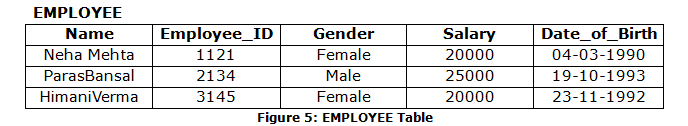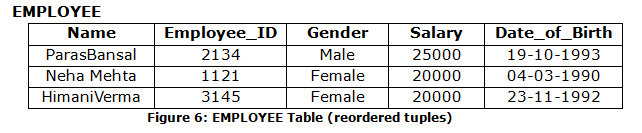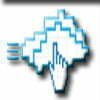Home » Online Computer Education » Learn online DBMS “What is Relational Database” Complete Lesson 5

# Learn online DBMS “What is Relational Database” Complete Lesson 5

Relational Database

Various types of databases were developed. One of them was relational database which was developed by E.F Codd at IBM in 1970. It is a collection of data items organized as a collection of relations where each relation corresponds to a table of values. A table consists of rows and columns. Each row in the table corresponds to a unique instance of data and each column is used to interpret the meaning of that data in each row. For example, consider EMPLOYEE table in Figure5. Each row in this table represents facts about a particular employee. The column names – Name, Employee_ID, Gender, Salary, Date_of_Birth – specify how to interpret the data in each row based on the column it lies in.In relational model,
• A row is called as a Tuple.
• A column is called as an Attribute.
• A table is called as a Relation.
• The data type of values in each column is called as the Domain.
• The number of attributes in a relation is called as the Degree of a relation.
• The number of rows in a relation is called as the Cardinality of a relation.
• Relation Schema R is denoted by R(A1,A2,A3…An) where R is the relation name and A1, A2,A3,….An is the list of attributes.
• Relation State is the set of tuples in the relation. A relation state r of relation schema R(A1, A2, …, An), denoted r(R) is a set of n-tuples
r = {t1, t2,…., tm}
wheren-tupleis the ordered list of values t = <v1, v2, …, vn>, where vi is in domain of Ai or is NULL. Here n is the degree of the relation and m is the cardinality of the relation.

Hence in Figure5,
• EMPLOYEE table is a Relation.
• There are three tuples in EMPLOYEE relation.
• Name, Employee_ID, Gender, Salary, Date_of_Birth – are attributes.
• The domain is a set of atomic (or indivisible) values. The domain of a database attribute is the set of all the possible values that attribute may contain. In order to specify a domain, we specify the data type of that
attribute. Following are the examples of domain of the EMPLOYEE relation:
a) Name – Set of character strings representing names of persons.
b) Employee_ID–Set of 4-digit numbers
c) Gender – male or female
d) Salary – Number
e) Date_of_Birth – Should have a valid date, month and year. The age of the employee must come out to be more than 18 and less than 65. Also the format should be dd-mm-yyyy.
• The degree of the EMPLOYEE relation is 5 as there are five attributes in this relation.
• The cardinality of the EMPLOYEE relation is 3 as there are three tuples in this relation.
• Relation Schema – EMPLOYEE (Name, Employee_ID, Gender, Salary, Date_of_Birth)
• Relation State –{<Neha Mehta, 1121,Female,20000,04-03-1990>,
<ParasBansal,2134,Male,25000,19-10-1993>,
<HimaniVerma,3145,Female,20000,23-11-1992>}

Few more characteristics of Relations:
1. Ordering of tuples is not important in a Relation. Thus the following relation in Figure 6is same as the relation in Figure 5.2. The ordering of attributes is also unimportant.
3. No two tuples of relation should be identical i.e. in any two tuplesvalue in at least one column must be different.
4. The value in each tuple is an atomic value (indivisible).
5. If the value of an attribute in a tuple is not known or not applicable or not available, a special value called nullis used to represent them.
For example consider the following cases:
• Unknown value:A person has a date of birth but it is unknown.
• Unavailable value: A person has a home phone but does not want it to be listed.
• Not applicable: College degree attribute would be NULL for a person who has no college degrees.
In all the above cases NULL value would be used.
A Relational DBMS (RDBMS) is a DBMS which is based on the relational model as discussed above. This is one of the most commonly used databases. Examples of RDBMS are – Oracle, MySOL, IBM DB2 etc.The main objective of this website is to provide quality study material to all students (from 1st to 12th class of any board) irrespective of their background as our motto is “Education for Everyone”. It is also a very good platform for teachers who want to share their valuable knowledge.